# Lepton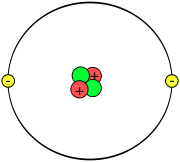Not-to-scale schematic of the classical view of a helium atom, showing two protons (red), two neutrons (green), and two electrons (yellow). Each electron belongs to the lepton group of elementary particles.

In particle physics, a lepton is one of the elementary (or fundamental) particles that are the building blocks of matter. Elementary particles are classified as fermions and bosons, and fermions are subdivided into leptons and quarks. A lepton is a fermion that does not experience the strong interaction (or strong nuclear force), which involves coupling with the bosons known as gluons. In other words, leptons are those fermions that "ignore" gluons. By comparison, quarks are fermions that couple with gluons to form composite particles such as protons and neutrons.

Leptons and quarks come in pairs, and in three generations. Everyday matter is composed of the first generation: two leptons, the electron and electron-neutrino; and two quarks, called Up and Down.

## Contents

As is the case for all fundamental particles, the lepton has properties of both a wave and a particle—it exhibits what is known as "wave-particle duality." The usual convention is to refer to such unified wave-particle fundamental entities as just "particles." The particle aspect is point-like even at scales thousands of times smaller than the proton size.

## Etymology

According to the Oxford English Dictionary, the name "lepton" (from Greek leptos) was first used by physicist Léon Rosenfeld in 1948:

Following a suggestion of Prof. C. Møller, I adopt—as a pendant to "nucleon"—the denomination "lepton" (from λεπτός, small, thin, delicate) to denote a particle of small mass. The name originated prior to the discovery in the 1970s of the heavy tau lepton, which is nearly twice the mass of a proton.

## Properties of leptons

As is the case for all fundamental particles, the lepton is a unified entity of wave and particle—the wave-particle duality of quantum physics. The wave "tells" the particle what to do over time, while the interactions of the particle "tell" the wave how to develop and resonate. The particle aspect is point-like even at scales thousands of times smaller than the proton size. The usual convention is to refer to such unified wave-particle fundamental entities as just 'particles'.

There are three known flavors of lepton: the electron, the muon, and the tau. Each flavor is represented by a pair of particles called a weak doublet. One is a massive charged particle that bears the same name as its flavor (like the electron). The other is a nearly massless neutral particle called a neutrino (such as the electron neutrino). All six of these particles have corresponding antiparticles (such as the positron or the electron antineutrino). All known charged leptons have a single unit of negative or positive electric charge (depending on whether they are particles or antiparticles) and all of the neutrinos and antineutrinos have zero electric charge. The charged leptons have two possible spin states, while only one helicity is observed for the neutrinos (all the neutrinos are left-handed, and all the antineutrinos are right-handed).

The masses of the leptons also obey a simple relation, known as the Koide formula, but at present this relationship cannot be explained.

When particles interact, generally the number of leptons of the same type (electrons and electron neutrinos, muons and muon neutrinos, tau leptons and tau neutrinos) remains the same. This principle is known as conservation of lepton number. Conservation of the number of leptons of different flavors (for example, electron number or muon number) may sometimes be violated (as in neutrino oscillation). A much stronger conservation law is the total number of leptons of all flavors, which is violated by a tiny amount in the Standard Model by the so-called chiral anomaly.

The couplings of the leptons to gauge bosons are flavor-independent. This property is called lepton universality and has been tested in measurements of the tau and muon lifetimes and of Z-boson partial decay widths, particularly at the SLC and LEP experiments.

### Quantum spin

Fermions and bosons are distinguished by their quantum spin and the type of quantum probability statistics they obey: Fermi-Dirac probability or Bose-Einstein probability, neither of which is like classical probability. (This is a rough illustration of the difference: (one) The probability of two classical coins coming up the same side—HH or TT—is 50 percent. (two) For two boson coins, the probability of such a pair is 100 percent. (three) For two fermion coins, the probability of a pair is exactly zero percent, it is forbidden, and you always get HT. Fermions are said to have quantum spin -½, giving them the odd property of having to be rotated 720° in order to get back to where you started. (A familiar example of this sort of behavior is the Moebius Strip.) Bosons have quantum spin -1, and take the usual 360° to rotate back to where they started.

## Table of the leptons

Name Symbol Electric charge (e) Mass (MeV/c2) Name Symbol Electric charge (e) Charged lepton / antiparticle Neutrino / antineutrino Electron / Positron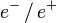$e^- \, / \, e^+$ −1 / +1 0.511 Electron neutrino / Electron antineutrino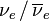$\nu_e \, / \, \overline{\nu}_e$ 0 < 0.0000022  Muon$\mu^- \, / \, \mu^+$ −1 / +1 105.7 Muon neutrino / Muon antineutrino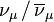$\nu_\mu \, / \, \overline{\nu}_\mu$ 0 < 0.17  Tau lepton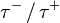$\tau^- \, / \, \tau^+$ −1 / +1 1777 Tau neutrino / Tau antineutrino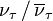$\nu_\tau \, / \, \overline{\nu}_\tau$ 0 < 15.5 

Note that the neutrino masses are known to be non-zero because of neutrino oscillation, but their masses are sufficiently light that they have not been measured directly as of 2007. The names "mu" and "tau" seem to have been selected due to their places in the Greek alphabet; mu is seven letters after epsilon (electron), whereas tau is seven letter after mu.